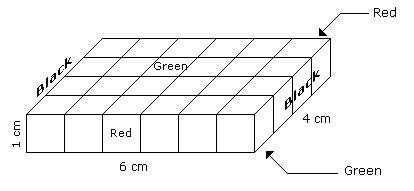# Verbal Reasoning - Cube and Cuboid - Discussion

Discussion Forum : Cube and Cuboid - Cube and Cuboid 1 (Q.No. 4)
Directions to Solve

The following questions are based on the information given below:

1. A cuboid shaped wooden block has 6 cm length, 4 cm breadth and 1 cm height.
2. Two faces measuring 4 cm x 1 cm are coloured in black.
3. Two faces measuring 6 cm x 1 cm are coloured in red.
4. Two faces measuring 6 cm x 4 cm are coloured in green.
5. The block is divided into 6 equal cubes of side 1 cm (from 6 cm side), 4 equal cubes of side 1 cm(from 4 cm side).

4.
How many cubes will have green colour on two sides and rest of the four sides having no colour ?
12
10
8
4
Explanation:There are 16 small cubes attached to the outer walls of the cuboid.

Therefore remaining inner small cubes will be the cubes having two sides green coloured.

So the required number = 24 - 16 = 8

Discussion:
4 comments Page 1 of 1.

Nishanth said:   4 years ago
There are 6 x 4 x 1 = 24,

Number of edge cubes are = 16.
so, 24 - 16 = 8.

Sofia zoe said:   6 years ago
Leaving edge cubes just count the number of cubes in the middle.
The total number of cubes are 6*4*1=24.
Number of edge cubes are=16,
24- 16 = 8.